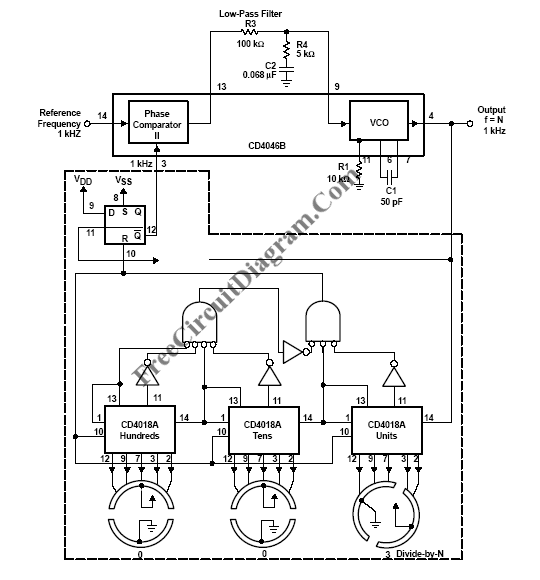# Frequency SynthesizerA PLL system can be used to build a frequency-selective frequency multiplier by inserting a frequency divider inside the feedback path between the VCO output and the phase error detector input. Here is the schematic diagram of a low-frequency synthesizer with a programmable three decades divider circuit.The frequency-divider modulus N can vary from 3 to 999, in single steps increment. In locked condition, the signal and comparator inputs are at the same frequency, and f = N × 1 kHz. The result is a frequency synthesizer with 3 kHz to 999 kHz range in 1-kHz increments, which is programmable by the switch position of the divide-by-n counter.

Because it shouldn’t lock on harmonics of the signal-input reference frequency,  ape comparator II is used for this application (phase comparator I does lock on harmonics). Since the active factor of the output of the divide-by-n frequency divider is not 50%, phase comparator II lends itself directly to this application. Phase comparator II set the VCO to cover a range of 0 MHz to 1.1 MHz. The LPF for this application is a two-pole, tag-lead filter that enables faster locking for step changes in frequency. [Schematic diagram source: Texas Instruments Application Report]